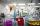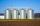Hyperbola equation

Find the hyperbola equation with the center of S [0; 0], passing through the points:
A [5; 3] B [8; -10]

Result

f = (Correct answer is: f = 7 * x^2 - 3y^2 = 148)Solution:Leave us a comment of this math problem and its solution (i.e. if it is still somewhat unclear...):Be the first to comment!To solve this verbal math problem are needed these knowledge from mathematics:

Looking for help with calculating roots of a quadratic equation? Do you have a linear equation or system of equations and looking for its solution? Or do you have quadratic equation?

Next similar math problems:

1. Evaluation of expressionsIf a2-3a+1=0, find (i)a2+1/a2 (ii) a3+1/a3Find the roots of the quadratic equation: 3x2-4x + (-4) = 0.
3. Geometric seqFind the third member of geometric progression if a1 + a2 = 36 and a1 + a3 = 90. Calculate its quotient.
4. Ball gameRichard, Denis and Denise together scored 932 goals. Denis scored 4 goals over Denise but Denis scored 24 goals less than Richard. Determine the number of goals for each player.
5. DiscriminantDetermine the discriminant of the equation: ?
6. Reciprocal equation 2Solve this equation: x + 5/x - 6 = 4/11
7. Square root 2If the square root of 3m2 +22 and -x = 0, and x=7, what is m?
8. RootsDetermine the quadratic equation absolute coefficient q, that the equation has a real double root and the root x calculate: ?
9. Solve 3Solve quadratic equation: (6n+1) (4n-1) = 3n2
10. EquationEquation ? has one root x1 = 8. Determine the coefficient b and the second root x2.
11. GP - three membersThe second and third of a geometric progression are 24 and 12(c+1) respectively, given that the sum of the first three terms of progression is 76 determine value of c
12. Solve equationsolve equation: ?
13. Crystal waterThe chemist wanted to check the content of water of crystallization of chromic potassium alum K2SO4 * Cr2 (SO4) 3 * 24 H2O, which was a long time in the laboratory. From 96.8 g of K2SO4 * Cr2 (SO4) 3 * 24 H2O prepared 979 cm3 solution of base. SQuadratic equation ? has roots x1 = -26 and x2 = -86. Calculate the coefficients b and c.In the three warehouses, a total of 70 tons of grain was stored. In the second warehouse was stored 8.5t less and in the third 3.5t more than in the first. How many tons of grain was stored in each warehouse?Solve two equations with two unknowns: 400x+120y=147.2 350x+200y=144We want to prove the sentence: If the natural number n is divisible by six, then n is divisible by three. From what assumption we started?